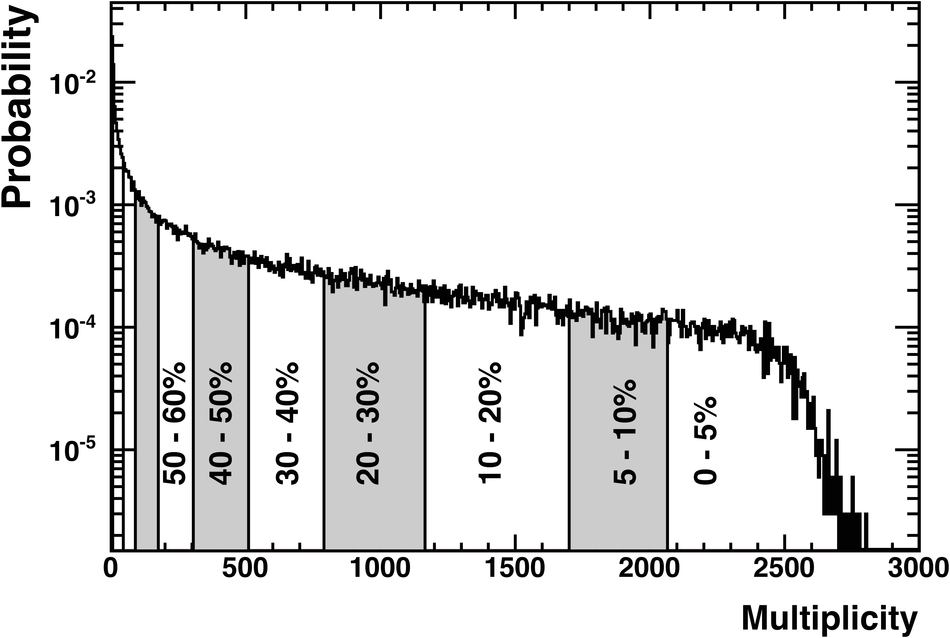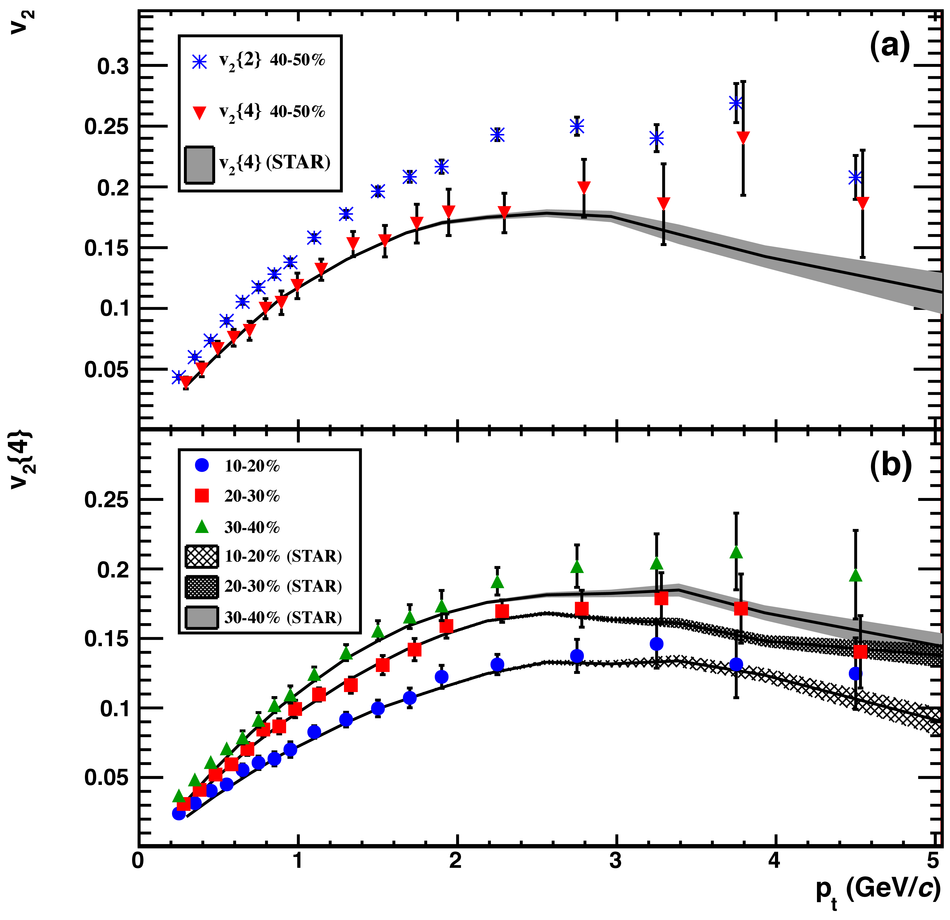# Elliptic flow of charged particles in Pb-Pb collisions at $\sqrt{s_{\rm NN}}$ = 2.76 TeV

We report the first measurement of charged particle elliptic flow in Pb-Pb collisions at $\sqrt{s_{\rm NN}}$ = 2.76 TeV with the ALICE detector at the CERN Large Hadron Collider. The measurement is performed in the central pseudorapidity region (|$\eta$|< 0.8) and transverse momentum range 0.2 < $p_{\rm T}$ < 5.0 GeV/$c$. The elliptic flow signal v$_2$, measured using the 4-particle correlation method, averaged over transverse momentum and pseudorapidity is 0.087 $\pm$ 0.002 (stat) $\pm$ 0.004 (syst) in the 40-50% centrality class. The differential elliptic flow v$_2(p_{\rm T})$ reaches a maximum of 0.2 near $p_{\rm T}$ = 3 GeV/$c$. Compared to RHIC Au-Au collisions at 200 GeV, the elliptic flow increases by about 30%. Some hydrodynamic model predictions which include viscous corrections are in agreement with the observed increase.

Figures

## Figure 1

 The uncorrected multiplicity distribution of charged particles in the TPC $(|\eta|< 0.8)$. The centrality bins used in the analysis are shown and the cumulative fraction of the total events is indicated in percent The bins 60-70% and 70-80% are not labeled.## Figure 2

 a) $v_2(\pt)$ for the centrality bin 40-50% from the 2- and 4-particle cumulant methods for this measurement and for Au--Au collisions at $\snn~=~200~$GeV b) $v_2${4}($\pt$) for various centralities compared to STAR measurements. The data points in the 20-30% centrality bin are shifted in $p_{\rm t}$ for visibility.## Figure 3

 Elliptic flow integrated over the $p_{\rm t}$ range $0.2< p_{\rm t}< 5.0$~GeV/$c$, as a function of event centrality, for the 2- and 4-particle cumulant methods, a fit of the distribution of the flow vector, and the Lee-Yang Zeroes method For the cumulants the measurements are shown for all charged particles (full markers) and same charge particles (open markers) Data points are shifted for visibility RHIC measurements for Au-Au at $\snn~=~200~$GeV, integrated over the $p_{\rm t}$ range $0.15< p_{\rm t}< 2.0~$GeV/$c$, for the event plane $v_2\{{\rm EP}\}$ and Lee-Yang Zeroes are shown by the solid curves## Figure 4

 Integrated elliptic flow at 2.76 TeV in Pb-Pb 20-30% centrality class compared with results from lower energies taken at similar centralities [40,43]